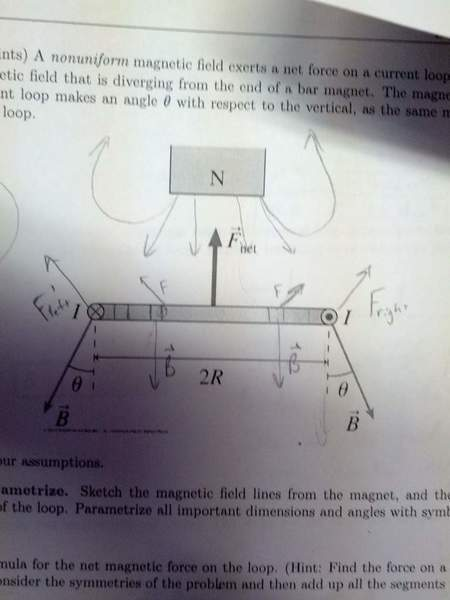# Current Loop in a nonuniform magnetic field

• PEZenfuego

## Homework Statement

A nonuniform magnetic field exerts a net force on a current loop of radius R. The figure shows a magnetic field that is diverging from the end of a bar magnet. The magnetic field B at the position of the current loop makes an angle θ with respect to the vertical, as the same magnitude at each point on the current loop. (I know that I need to solve in terms of R, I, B, and θF=IlxB

F=IlBsinθ

τ=μxB

τ=μBsinθ

## The Attempt at a Solution

I fought the urge to use the force equation after substitution 2∏r for l. Instead I examined the force on one small segment and planned to integrate. The length would be in terms of arc length Δs. The I would be a constant. It seems that the problem indicates that the magnetic field B is constant (surely that is an assumption because the distance from the magnet was not indicated, right?) I also thought that the angle should be the only thing changing (I doubt this is a double integral problem). The subscript i indicates that this is for some segment i.

So

Fi=IΔsBsinθi

The first thing that jumps out at me is that we don't have the necessary Δθ, but we do have Δs. Δs=ΔθR, but this is for a different θ, right? So, here is where I got lost and thought that something was wrong.

Next I tried relating it with torque.

τ=μBsinθ=FR
τ=IABsinθi
τ=I((2∏R^2)/(θ)/2∏B))sinθi=FR

But here again we have a different θ value, correct?

I would very much appreciate some help. Thank you!

What are you supposed to solve for?

Net force

Use the differential form of your F equation above, which is
d F = I d l x B for the force on an element d l of the loop.

Then integrate around the loop - an easy integration since the force is constant everywhere around the loop.

I know that the answer is to be 2πRIBsinθ, but I don't see how or why.

dF=IdlxB is the same as saying dF=IΔsxB or dF=IBsinθΔs integrating yields

F=IBssinθ or F=2πRsinθ.

But doesn't the value of theta change for each segment?

Oh wait! No, it doesn't. It should remain constant as the ring has rotational symmetry. Am I correct in my reasoning here?

It is important to note that in the formula F = I B Δs sinθ, θ is not the same θ as given in the diagram. Remember, in the formula, sinθ is coming from a cross product of Δs and B.

I know that the answer is to be 2πRIBsinθ, but I don't see how or why.

dF=IdlxB is the same as saying dF=IΔsxB or dF=IBsinθΔs integrating yields

F=IBssinθ or F=2πRsinθ.

But doesn't the value of theta change for each segment?

Oh wait! No, it doesn't. It should remain constant as the ring has rotational symmetry. Am I correct in my reasoning here?

That is correct! And take note of what tsny says about theta. Keep track of angles when you take your cross-product!